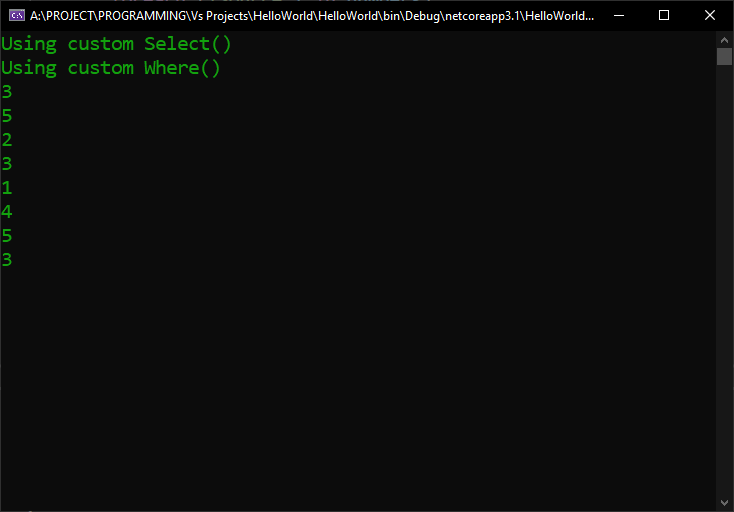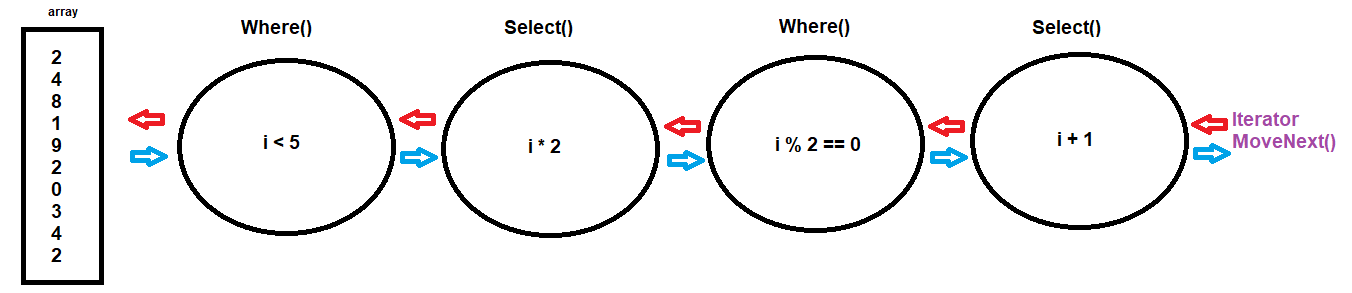Saturday, September 23, 2023 22:14

## LINQ execution workflow

In the previous lesson, I talked about the fact that LINQ actually delays the execution of its constituent queries up until the very last moment, when we actually need the data. What was not as obvious at the time was the order in which the LINQ queries are executed.

Let’s take the example we ended up with and extend it a little bit:

So, we have a simple array of integer numbers, which we run through a LINQ pipeline in which we first take the elements that are less than five, then we multiply each of the results by two, then we filter the results yet again by even numbers, and finally, we add one to the elements of the results.

In a normal way of understanding C# program execution workflows, nested calls that depend on the result of the previous method are thought of as going from left to right. If we have three methods that call on each other’s result, like this: methodA().methodB().methodC();, it comes to us naturally to imply that the execution starts with methodA(), which pushes the result onto methodB(), which relays its result to methodC(), in this particular order.

With LINQ, this is not at all true. In the previous lesson, when we used custom built Select() and Where() functions, we had these codes:So, even though Where() is called first, and Select() is called after, receiving its data from the result the Where() function produces, you can clearly see that the Select() function is actually the first called and executed.

Why is that?

It all has to do with the concept of deferred execution, explained in the previous lesson, and the fact that LINQ calls are actually just extension method calls, which we learned that are nothing more than static method calls. In other words, the above LINQ query could be written as Enumerable.Where(Enumerable.Select(numbers, i => i + 1), i => i < 5);. I know, it looks horrible, but what is clear from that is the fact that these calls take as their parameters the result of calling the next method, which also takes as parameter the result of calling the next method, and so on. Since no call can be made until all the parameters are resolved, that means that the CLR needs to execute the deepest method called as parameter, which is the innermost Select(). This is the reason why Select() is called first, because its result is needed as a parameter for the Where().

This could be reasoned and deducted even from the LINQ style of calls: the Select() function is the one that outputs the results when we iterate the LINQ query. But, where does Select() take its source of data, that it needs to select? From the Where() function. And where does the Where() function takes its data source? From the array.

So, when we iterate the above LINQ query, the call needs to travel two ways, actually. The enumerator’s MoveNext() asks a number from the Select() function, which asks it from the Where(), which takes it from the array; then, the Where() function does the checking if the number is less than 5, and if it is, it relays it to the Select(), which adds 1 to it, and gives back to the iterator. In simple words, the iterator asks for data from the query output, which has to travel all the way to the array data source, and back again, through all the filters, back to the iterator.

In the example with which we started this lesson, this would translate to:where i would be constantly replaced by all the numbers in the array, sequentially.

This implies that: for the first number in the array, the call travels from the iterator to the array, asks the number 2, returns to the first Where(), which checks if the number is less than 5. Since it is, it relays it to the Select(), which multiplies it by 2, and relays it to the second Where(), which checks if it’s an even number, and because 10 is, it relays it to the last Select(), which adds 1 to it, resulting in the number 11 being returned to the iterator.

Then, the iterator asks for the next number, and the whole process repeats, this time with the number 4 from the array.

When it reaches the element 8 in the array, the check of being less than 5 in the first Where() fails, so, the number is not propagated forward, back to the Select(); instead, Where() asks again for another number, in our case, 1, which does satisfy the condition of being less than 5, which is multiplied by 2, and so on, and so forth.

So, LINQ queries are efficient due to deferred execution. Nothing executes until we actually need the results. On the other hand, LINQ queries need to have a two way trip for each of the elements of the queries, which sometimes can affect performance a tiny bit, especially on very large amount of data.